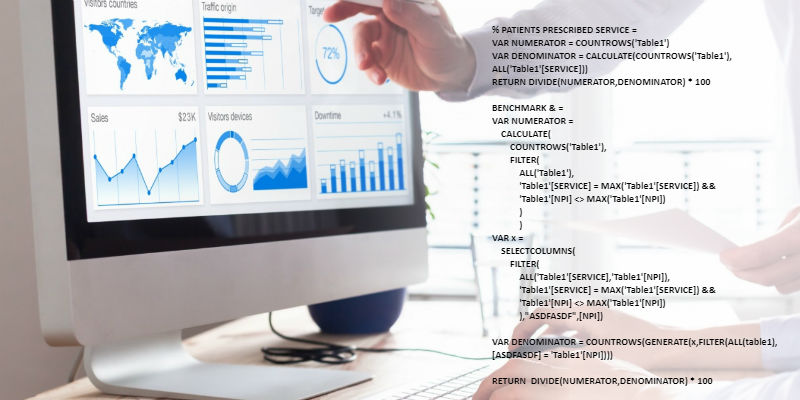# DAXDuración
10-20 horas

The DAX data modeling language developed by Microsoft is probably the biggest difference between Power BI and the rest of the competitors in the Business Intelligence industry. DAX is a library of functions and operators that can be combined for data modeling in Power BI, Azure Analysis Services, SQL Server Analysis Services (SSAS) and Power Pivot for Excel.

In this introductory course we will begin by justifying the need for a tool of this type for the creation of calculated columns and the modeling of measures, we will review some of the more complex concepts related to the use of DAX -mainly the so-called "context"- and we will review some of the most frequently used DAX functions as we apply them to real scenarios of incremental complexity.

### Content:

• Justification of the need for data modeling languages
• Create calculated columns
• Measure creation
• Context
• Introduction to DAX functions
• Date and time functions
• Time Intelligence functions
• Filtering functions
• Information functions
• Logical functions
• Mathematical and trigonometric functions
• Parent-child hierarchy functions
• Statistical functions
• Text functions
• Other functions